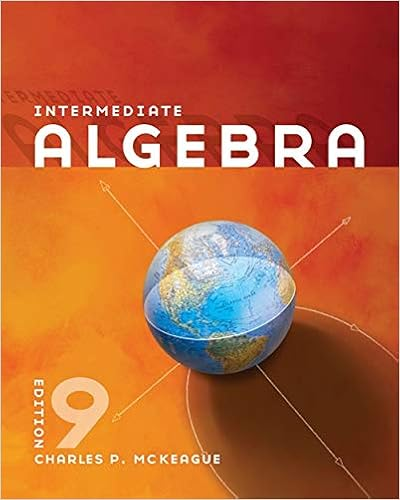# Pt the table below gives the number of calories

• Homework Help
• 4
• 100% (11) 11 out of 11 people found this document helpful

This preview shows page 3 - 4 out of 4 pages.

##### We have textbook solutions for you!
The document you are viewing contains questions related to this textbook.The document you are viewing contains questions related to this textbook.
Chapter 6 / Exercise 71
Intermediate Algebra
MckeagueExpert Verified
12. (1 pt) The table below gives the number of calories burned per minute for someone roller-blading, as a function of the person’s weight in pounds and speed in miles per hour [from the August 28,1994, issue of Parade Magazine ]. calories burned per minute weight \ speed 8 9 10 11 120 4.2 5.8 7.4 8.9 (1 pt) Let f be the linear function f ( x , y ) = c + mx + ny , where c , m , n are constants and n 6 = 0. Note: For all parts of this problem, your answers must be in ex- panded form–that is, may not contain any parentheses–and may involve the constants c , m , n and k. (a) Find and expand the equation of the contour that goes through the value f ( x , y ) = k . 200 7.9 9.5 11.1 12.6 (a) Suppose that a 140 lb person and a 180 person both go 10 miles, the first at 11 mph and the second at 10 mph. How many calories does the 140 lb person burn? How many calories does the 180 lb person burn? (b) We might also be interested in the number of calories each person burns per pound of their weight. How many calories per pound does the 140 lb person burn? How many calories per pound does the 180 lb person burn? 13. (1 pt) Find a possible equation for the linear function g ( x , y ) shown in the graph below: g ( x , y ) = 14. (1 pt) Find the equation for a linear function f ( x , y ) with the values given in the table below: x \ y - 1 0 1 2 0 -3.5 -2 -0.5 1 15. (1 pt) Let f be the linear function f ( x , y ) = c + mx + ny , where c , m , n are constants and n 6 = 0. Note: For all parts of this problem, your answers must be in ex- panded form–that is, may not contain any parentheses–and may involve the constants c , m , n and k. (a) Find and expand the equation of the contour that goes through the value f ( x , y ) = k .
##### We have textbook solutions for you!
The document you are viewing contains questions related to this textbook.The document you are viewing contains questions related to this textbook.
Chapter 6 / Exercise 71
Intermediate Algebra
MckeagueExpert Verified
•••